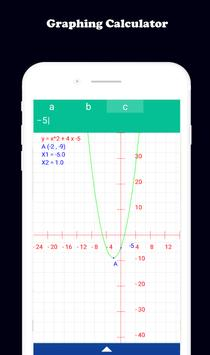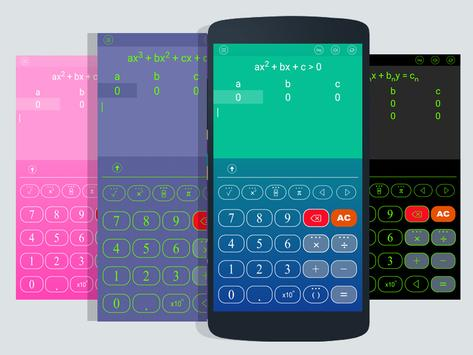HiEdu Scientific Calculator : Fx-570vn Plus APK Free Download
Version:  3.9.4

### Application Description:

HiEdu Scientific Calculator : Fx-570vn Plus : A simple yet powerful calculator that includes standard, scientific, and programmer modes, as well as a unit converter, Math, Physics and Chemistry Formulas, graph and solve the equations . It's the perfect tool to add up a bill, convert measurements in a recipe or other project and It is a very useful tool for education help complete complex math, algebra, or geometry problems.

EY FEATURES
Scientific Calc
- It is a scientific calculator supports most of the features of real calculators such as Casio fx570ms, fx570esplus, 570vnplus ...
- Natural Display makes it possible to input and display fractions and certain functions (log, x2, x3, x^, ), ", xˆ’1, 10^, e^ , d/dx, خ, Abs ...) just as they are written in your textbook.
- Undo when you miss a mistake.
- Save history, Select a calculation in list history and edit it.
- Create favorite calculations that make calculations faster.

Standard Calc
- This is a perfect tool for daily calculations. With memory functions similar to a small handheld calculator
- Save history, Select a calculation in list history and edit it.

Mathematics formulas
- This app has 1000+ math formula and more to come.
- Now no need to make paper notes to remember mathematics formulas just have this app put all the formulas on your favourite phones.
- you'll find formulas very simply explained in app with necessary figures will help you to understand very easily.

Physics formulas
- The application is a physical handbook. Contains most physical formulas for students and undergraduate.help users quickly refer to any Physics formulas for their study and work.This app displays most popular as well as advanced formulas in seven categories:
Mechanics
Electricity
Thermal physics
Periodic motion
Optics
Atomic physics
Constants

Chemical reactions
- Allows to discover chemical reactions and to solve the chemical equations with one and several unknown variables.

Programmers
- Can convert numbers between different number bases (2/8/10/16).
- Display shows numbers in binary, hexadecimal, octal and decimal.
- Input can be in binary, hexadecimal, octal or decimal.

Graphing calc
- Easily graph functions, solve equations, find special points of functions.

Solve the equations
- Linear equation for degree one,quadratic equation for degree two,cubic equation for degree three, System of linear equations.

Converter
- Available unit conversions include:
Currency (US dollar, CDN dollar, pound, peso, etc)
Temperature (celsius, fahrenheit, kelvin, etc)
Length (kilometer, miles, meter, yard, feet, etc)
Mass/Weight (kilogram, pound, ounce, ton, stone, etc)
Speed (km/h, mph, knot, etc)
Area (square kilometer, square mile, hectare, acre, etc)
Cooking Volume (teaspoon, tablespoon, cup, pint, quart, ounce, etc)
Pressure (kilopascal, bar, PSI, etc)
Power (watt, kilowatt, horsepower, etc)
Energy (joule, calorie, BTU, etc)
Time (year, month, day, hour, second, etc)
Fuel Consumption (miles per gallon, liters per 100km, etc)
Digital Storage (bit, byte, megabytes, gigabytes, etc)

## Conclusion

HiEdu Scientific Calculator : Fx-570vn Plus was filed under the Education category and was reviewed in softlookup.com and receive 5/5 Score.
HiEdu Scientific Calculator : Fx-570vn Plus has been tested by our team against viruses, spyware, adware, trojan, backdoors and was found to be 100% clean. We will recheck HiEdu Scientific Calculator : Fx-570vn Plus when updated to assure that it remains clean.

### HiEdu Scientific Calculator : Fx-570vn Plus user Review

Please review HiEdu Scientific Calculator : Fx-570vn Plus application and submit your comments below. We will collect all comments in an effort to determine whether the HiEdu Scientific Calculator : Fx-570vn Plus software is reliable, perform as expected and deliver the promised features and functionalities.